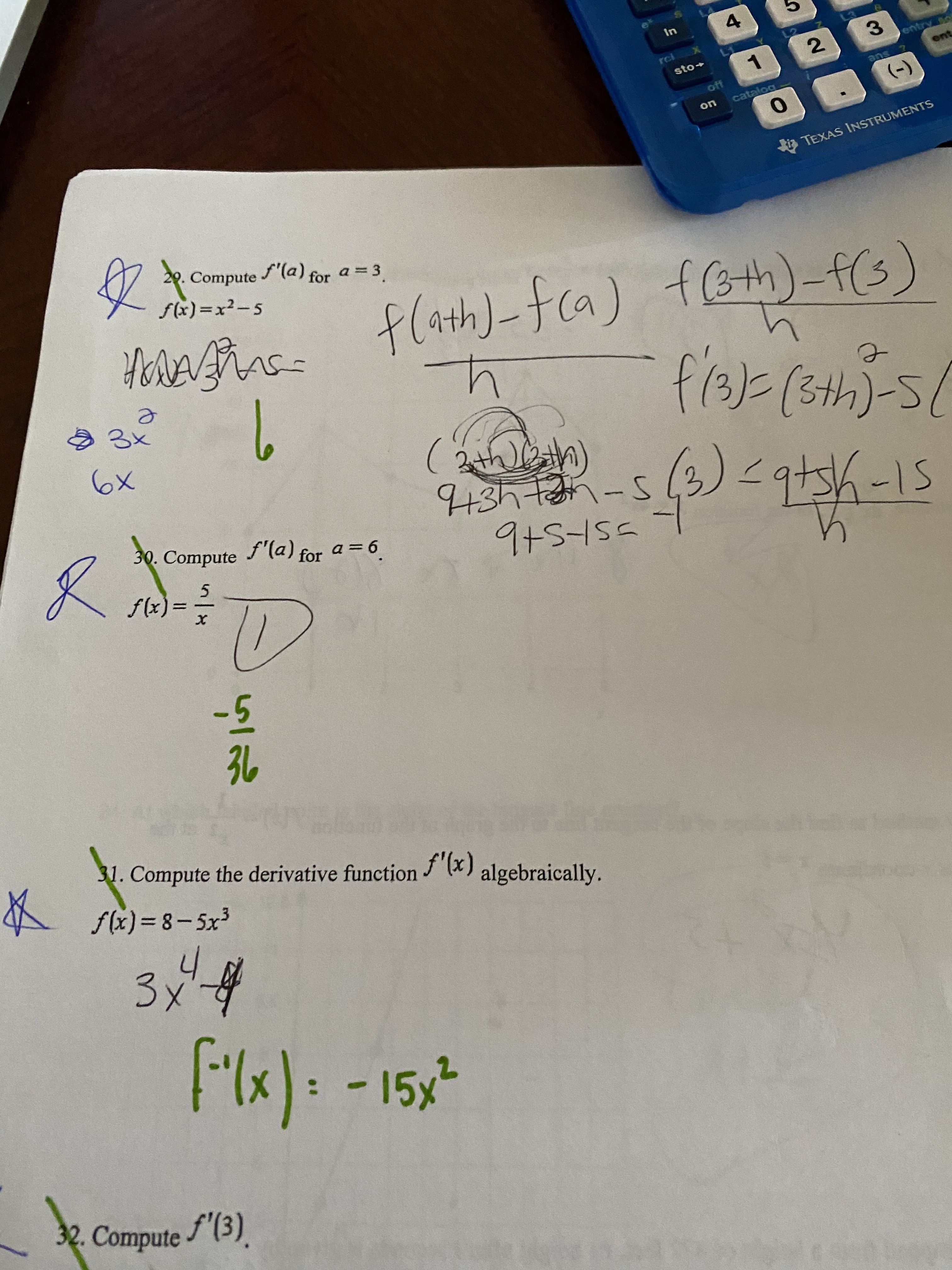# 4Inentry soentreisto+ansoff(-)catalogoni TEXAS INSTRUMENTS29. Compute(a) fora= 3sx) =x²-5flath)-fca) f(3th)-f(s)HorARA=f/3)=(3th)-5L9 3x6x2th)43htan-s30. Compute-15f'(a) for a= 6.f(x):-53631. Compute the derivative function "(x)A slx) =8- 5x?algebraically.3x-4f"lx): -15x32. Compute (3).3.6.2]

Question
1 views

question 31help_outlineImage Transcriptionclose4 In entry so ent rei sto+ ans off (-) catalog on i TEXAS INSTRUMENTS 29. Compute (a) for a= 3 sx) =x²-5 flath)-fca) f(3th)-f(s) HorARA= f/3)=(3th)-5L 9 3x 6x 2th) 43htan-s 30. Compute -15 f'(a) for a= 6. f(x): -5 36 31. Compute the derivative function "(x) A slx) =8- 5x? algebraically. 3x-4 f"lx): -15x 32. Compute (3). 3. 6. 2] fullscreen
check_circle

Step 1

Consider the given function.

Step 2

Formula used:

...

### Want to see the full answer?

See Solution

#### Want to see this answer and more?

Solutions are written by subject experts who are available 24/7. Questions are typically answered within 1 hour.*

See Solution
*Response times may vary by subject and question.
Tagged in

### Other